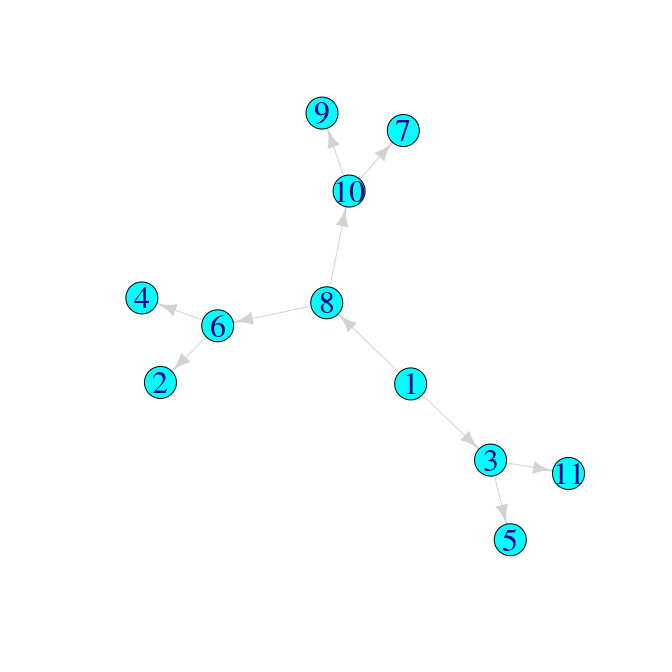treespace worked example: Transmission trees

2019-04-07

This vignette demonstrates the use of treespace to compare a collection of transmission trees as proposed in Kendall et al. 2018.

First we load the package treespace:

library(treespace)

treespace contains three functions for handling and comparing transmission trees:

1. findMRCIs() which takes a “who infected whom matrix” (the information about infectors and infectees; more on this below) and outputs:
• sourceCase: the number of the node which is the source case, i.e. the common infector of all cases (outputs a warning if there is more than one source case).

• mrcis: a matrix where, for each pair of individuals i and j, the entry (i,j) is the node number of their MRCI. Note that if i infected j then this entry is i itself.

• mrciDepths: a matrix where, for each pair of individuals i and j, the entry (i,j) is the depth of their MRCI, defined as the number of edges from the source case. The source case has depth zero, its direct infectees have depth 1, and so on.

1. wiwTreeDist() which takes a list of mrciDepths matrices and computes the distances between them. You have to supply the list of sampled cases in which you are interested, and then it takes the Euclidean distance between each pair of matrices restricted to the sampled cases (and written long-hand, as a vector)

2. wiwMedTree() which takes a list of mrciDepths matrices, the list of sampled cases, an optional list of weights, and outputs the median transmission tree

Examples

We define a “who infected whom matrix” as a matrix of two columns, where the first represents the infectors and the second represents their infectees. For example, a simple transmission chain could be represented like this:

tree1 <- cbind(Infector=1:5,Infectee=2:6)
tree1
##      Infector Infectee
## [1,]        1        2
## [2,]        2        3
## [3,]        3        4
## [4,]        4        5
## [5,]        5        6

This can be easily visualised as a transmission chain using graph plotting packages such as igraph or visNetwork:

library(igraph)
# set plotting options:
igraph_options(vertex.size=15,
vertex.color="cyan",
vertex.label.cex=2,
edge.color="lightgrey",
edge.arrow.size=1)

tree1graph <- graph_from_edgelist(tree1)
plot(tree1graph)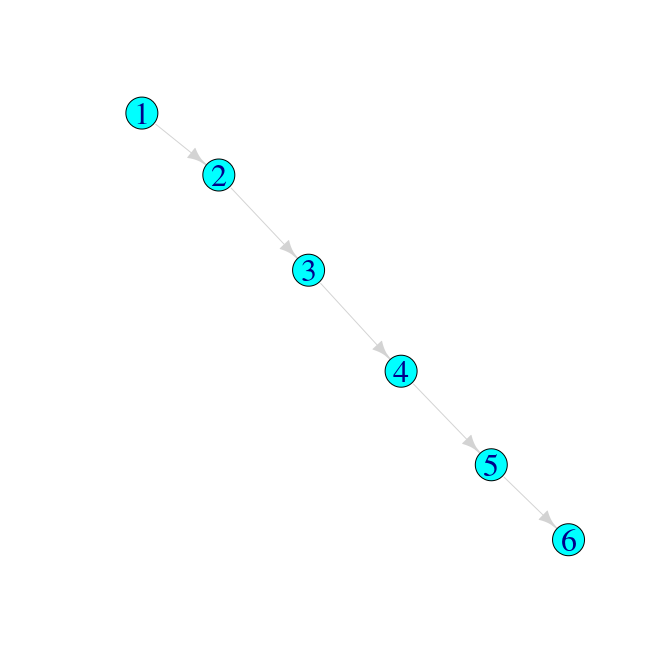Applying the function findMRCIs gives the following:

findMRCIs(tree1)
## $sourceCase ##  1 ## ##$mrcis
##   1 2 3 4 5 6
## 1 1 1 1 1 1 1
## 2 1 2 2 2 2 2
## 3 1 2 3 3 3 3
## 4 1 2 3 4 4 4
## 5 1 2 3 4 5 5
## 6 1 2 3 4 5 6
##
## \$mrciDepths
##   1 2 3 4 5 6
## 1 0 0 0 0 0 0
## 2 0 1 1 1 1 1
## 3 0 1 2 2 2 2
## 4 0 1 2 3 3 3
## 5 0 1 2 3 4 4
## 6 0 1 2 3 4 5

Comparing three simple trees

Suppose we had other hypotheses for the transmission tree which describes who infected whom amongst these six cases:

##      Infector Infectee
## [1,]        1        2
## [2,]        5        3
## [3,]        2        4
## [4,]        2        5
## [5,]        3        6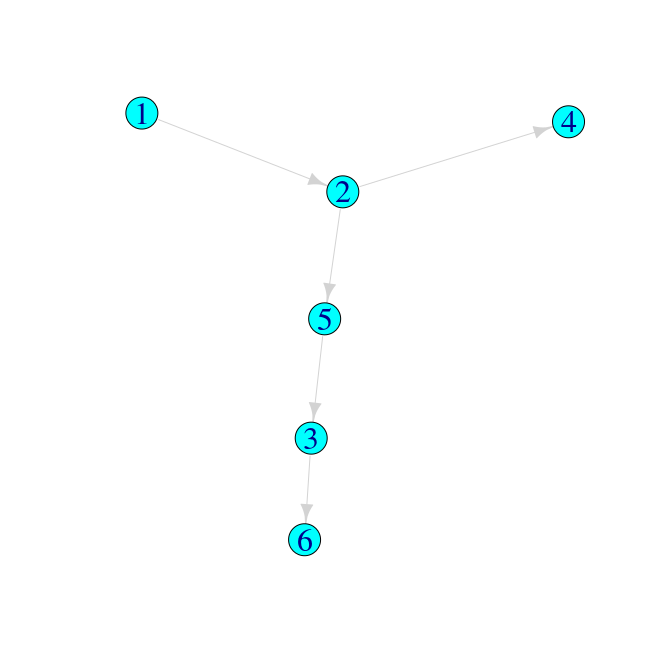##      Infector Infectee
## [1,]        2        1
## [2,]        2        3
## [3,]        2        4
## [4,]        2        6
## [5,]        6        5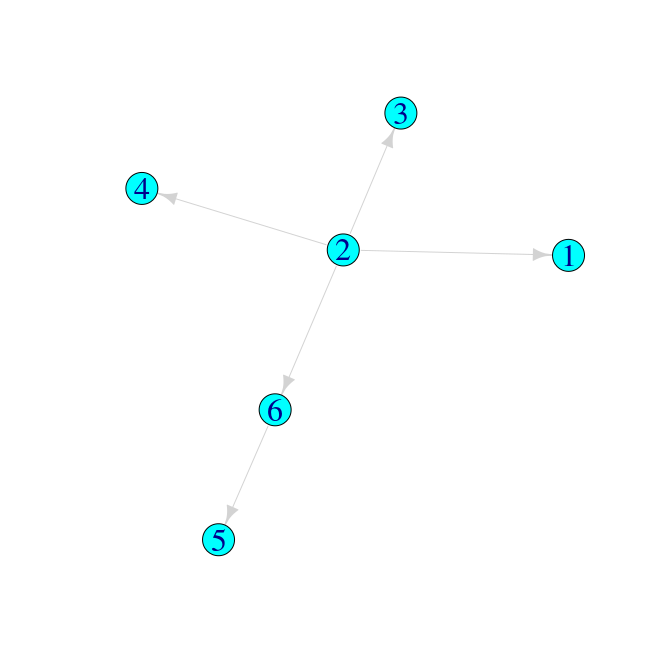Then we can use treespace functions to make the following comparisons:

## []
##   1 2 3 4 5 6
## 1 0 0 0 0 0 0
## 2 0 1 1 1 1 1
## 3 0 1 2 2 2 2
## 4 0 1 2 3 3 3
## 5 0 1 2 3 4 4
## 6 0 1 2 3 4 5
##
## []
##   1 5 2 3 4 6
## 1 0 0 0 0 0 0
## 5 0 2 1 2 1 2
## 2 0 1 1 1 1 1
## 3 0 2 1 3 1 3
## 4 0 1 1 1 2 1
## 6 0 2 1 3 1 4
##
## []
##   2 6 1 3 4 5
## 2 0 0 0 0 0 0
## 6 0 1 0 0 0 1
## 1 0 0 1 0 0 0
## 3 0 0 0 1 0 0
## 4 0 0 0 0 1 0
## 5 0 1 0 0 0 2
##        1      2
## 2  6.557
## 3 11.000  7.211

If we had only sampled cases 4, 5 and 6, so that “1”, “2” and “3” could be regarded as arbitrary names of inferred, unsampled cases, we would compute:

##       1     2
## 2 5.568
## 3 9.487 5.568

which substantially changes the measures of similarities and differences between the trees.

Comparing many trees using an MDS plot

Finally, we demonstrate comparing a larger set of transmission trees and finding the median:

Now that we have a pairwise distance matrix we can use multidimensional scaling (MDS) to view the relative distances between the trees in a 2D projection. We will colour the points in the projection by the “depth” of the corresponding tree, and use symbols to indicate the source case. For “depth” here we simply use the mean of each “mrciDepths” matrix.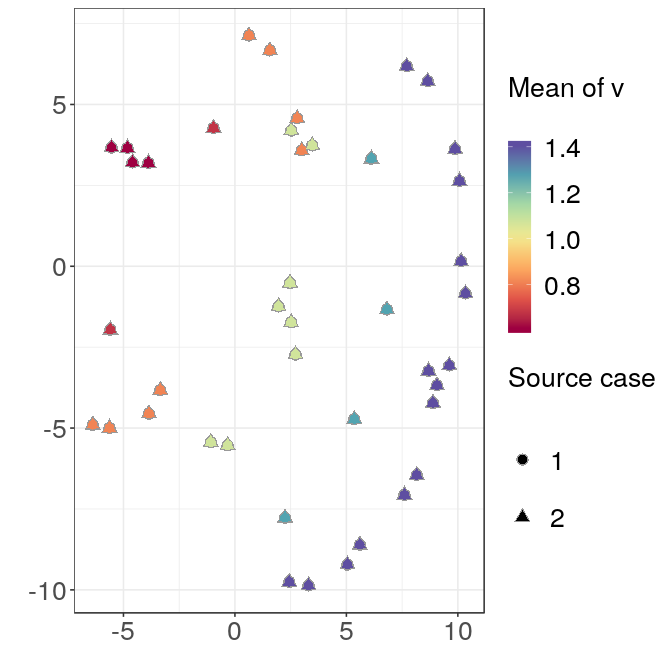The symmetry in the plot corresponds to the different source cases, and the trees are also clearly separated by depth.

Median trees

If our transmission trees corresponded to real data it could be meaningful to find a single representative tree. To find the geometric median tree(s) from a collection, we use the function wiwMedTree:

This returns a list with components:

##  "centre"    "distances" "mindist"   "median"
• centre is the mean vector (which may not necessarily correspond to a valid transmission tree with a single source case)

• distances gives the distance of each tree from the centre, as a vector

• mindist gives the minimum of these distances

• median gives the number(s) of the median tree(s)

Here the median trees are:

##     34   43   57   78   83   84  104  110  116  134  135  177  265  279
##   287  315  316  329  359  363  406  410  444  448  477  489  498  561
##   567  584  600  603  611  615  633  650  654  656  703  741  749  751
##   752  762  783  785  788  794  841  888  919  924  934  955  969 1000

The first one looks like this: